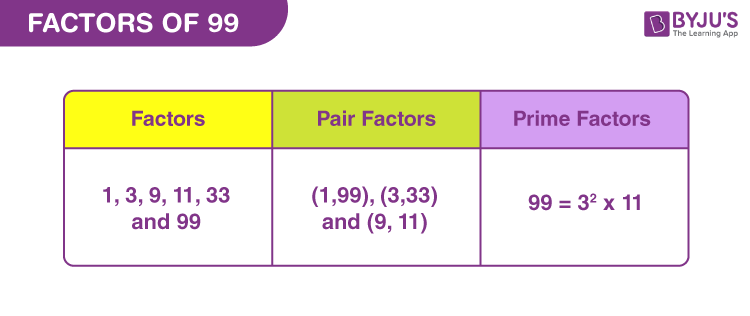# Factors of 99

Factors of 99 are the natural numbers that divide the original number, completely. So, if any factor divides the number 99, then there is no remainder left and a quotient is a whole number. Let us take an example of 8, whose factors are 1, 2, 4 and 8. Hence, if we divide 8 by 4 we get 2 as the result.

8 ÷ 4 = 2

Thus, there is no remainder and a quotient is a whole number.

In the same way, we can find the factors of 99 here in this article along with pair factors and prime factors. The pair factors result in the original number, when multiplied together and prime factors are the prime numbers that divide the original number, evenly.## How to Find Factors of 99?

Factors of 99 are the real numbers/integers that divide the original number, evenly, without leaving any remainder. 99 is a composite number, therefore, it will have more than two factors. We will start dividing 99 by the smallest natural number 1.

• 99 ÷ 1 = 99
• 99 ÷ 3 = 33
• 99 ÷ 9 = 11
• 99 ÷ 11 = 9
• 99 ÷ 33 = 3
• 99 ÷ 99 = 1

Thus, the factors of 99 are 1, 3, 9, 11, 33 and 99.

Remember: 99 is an odd number, therefore, it is not divisible by any even number.

## Pair Factors of 99

The product of pair factors of 99, will result in the original number, such that;

• 1 × 99 = 99
• 3 × 33 = 99
• 9 × 11 = 99

Therefore, the pair factors are (1, 99), (3, 33) and (9, 11).

As we can see, these were the positive pair factors. Similarly, if we consider negative pairs of factors, the multiplication of such two negative numbers will result in the original number.

• -1 × -99 = 99
• -3 × -33 = 99
• -9 × -11 = 99

Therefore, the negative pair factors are (-1, -99), (-3, -33) and (-9, -11).

## Prime Factorisation of 99

Prime factors of 99 are the prime numbers, that divide the original number, evenly. Alternatively, we can say, 99 is divisible by its prime factors. Now, we will use the prime factorisation method to find the prime factors.

Step 1: Dividing 99 by the smallest prime factor, which is 3, we get;

99/3 = 33

Step 2: Again divide 33 by the smallest prime factor, 3, to get;

33/33 = 11

Step 3: 11 is itself a prime factor, therefore, it is divisible by 11 only.

11/11 = 1

Therefore,

 Prime factorisation of 99 = 3 x 3 x 11 = 32 x 11

## Solved Examples

Q.1: 99 students have to go on a picnic. There are 33 seats available in the bus. How many students can be seated in one seat?

Solution: Given,

Number of students = 99

Number of seats in a bus = 33

Number of students sitting in a seat = 99/33 = 3

Q.2: What is the sum of all the factors of 99?

Solution: The factors of 99 are 1, 3, 9, 11, 33 and 99.

Sum = 1+3+9+11+33+99 = 156

Therefore, 156 is the required sum.

Q.3: What are the common factors of 11 and 99?

Answer: Let us write the factors of both the numbers.

11 → 1, 11 (Since 11 is a prime number)

99 → 1, 3, 9, 11, 33 and 99 (99 is a composite number)

Hence, we can see the common factors are 1 and 11.

## Frequently Asked Questions on Factors of 99

### 1. How many factors are there for number 99?

Answer: There are a total of six factors of 99. They are 1, 3, 9, 11, 33 and 99.

### 2. Is 99 a prime number?

Answer: No, 99 is not a prime number but a composite number because it has more than two factors.

### 3. What are the multiples of 99?

Answer: The first 10 multiples of 99 are 99, 198, 297, 396, 495, 594, 693, 792, 891 and 990.

### 4. What is 99 as a product of primes?

Answer: The prime factorisation of 99 is equal to:

99 = 3 x 3 x 11 = 32 x 11

### 5. What is the GCF of 99 and 100?

Answer: Factors of 99 are:1, 3, 9, 11, 33, 99

Factors of 100 are: 1, 2, 4, 5, 10, 20, 25, 50, 100

Therefore, the greatest common factor (GCF) of 99 and 100 is 1.